# Y4 Maths task- Thursday 30th April

Good morning everyone.

Well done for your work on symmetry over the last two days.

Today we are moving on to angles and lines.

Do you know any technical names for angles or lines already? ….

Parallel line– these lines run next to each other like train tracks and will never meet.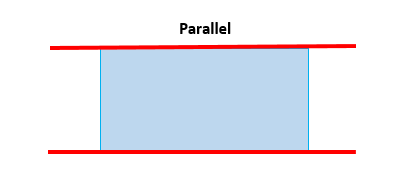Perpendicular lines– these lines will eventually meet at a point.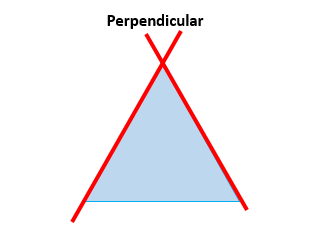Right angles– a right angle measures at 90 degrees. It looks like an L shape and is found in squares and rectangles.Obtuse angles– angles that are bigger than 90 degrees but smaller than 180 degrees (a straight line)acute angles– angles that are smaller than 90 degrees (I always remember that it is a cute, little baby angle!)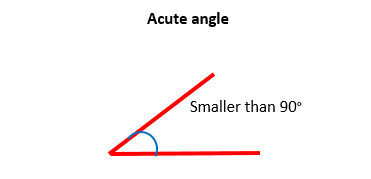reflex angles– normally measured on the outside of a shape, these angles are bigger than 180 degrees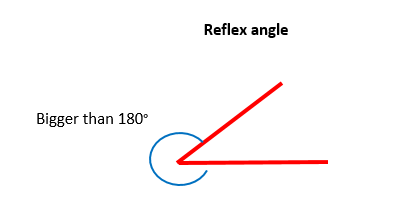All of these are on a sheet linked below for you to refer to.

I would like you to classify the lines and angles highlighted on the shapes using the information above.

I have loaded the sheet in two formats as I know some of you were struggling to access.

Line and angle classification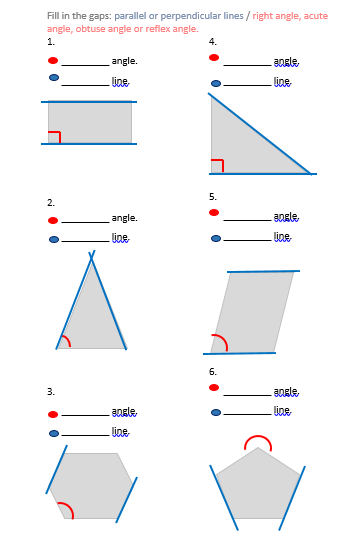Lines and angles

### 7 thoughts on “Y4 Maths task- Thursday 30th April”

•30th April 2020 at 10:21 am

1.
right angle
parallel
2.
acute angle
perpendicular
3.
acute angle
parallel
4.
right angle
perpendicular
5.
acute angle
parallel
6.
reflex angle
parallel

•30th April 2020 at 10:49 am

1.
Right angle
Parallel line
2.
Acute angle
Perpendicular line
3.
Obtuse angle
Parallel line
4.
Right angle
Perpendicular line
5.
Acute angle
Parallel line
6.
Reflex angle
Parallel line

•30th April 2020 at 10:55 am

Shapes
1
Right angle
Parallel line

2
Acute angle
Perpendicular lines

3
Obtuse angle
Parallel lines

4
Right angle
perpendicular lines

5
Acute angle
Parallel lines

6
Reflex angle
Perpendicular line

•30th April 2020 at 2:18 pm

1. Right angle, Parallel.
2. Acute angle, Perpendicular.
3. Obtuse angle, Parallel.
4. Right angle, Perpendicular.
5. Acute angle, Parallel.
6. Obtuse angle, Perpendicular.

•30th April 2020 at 2:40 pm

1.
right angle
parallel line
2.
acute angle
perpendicular lines
3.
obtuse angle
parallel llines
4.
right angle
perpendicular lines
5.
acute angle
parallel lines
6.
reflex angle
perpendicular lines

•30th April 2020 at 4:44 pm

1. Right angle and Parallel Lines
2. Acute angle and Perpendicular Lines
3. Obtuse angle and Parallel Lines
4. Right angle and Perpendicular Lines
5. Acute angle and Parallel Lines
6. Obtuse angle and Perpendicular Lines

•